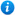# Dividing One and Two Digit Numbers by 100 - Year 4

Dividing One and Two Digit Numbers by 100 - Year 4

Year 4 maths programme of study - Number - fractions (including decimals)

• Find the effect of dividing a one- or two-digit number by 10 and 100, identifying the value of the digits in the answers as ones, tenths and hundredths

In this KS2 maths teaching resource pupils use a place value chart to see how the digits move when dividing by 100. It is an ideal teaching aid to use in a lesson covering the year 4 curriculum objective listed above. Content includes:

• Using a place value chart to divide one digit numbers by 100 activity and worksheet
• Reasoning and problem solving activity and worksheet
• Using a place value chart to divide two digit numbers by 100 activity and worksheet
• Using a Gattegno chart problem solving activity and worksheet
'Dividing One and Two Digit Numbers by 100 - Year 4' is editable so teachers can adapt the resource to meet their individual teaching needs.

Click on the images opposite to view 'Dividing One and Two Digit Numbers by 100 - Year 4' in full.

Our Price : £1.99 / 2 Credits## Get this resource as part of a bundle and save up to 55%## Year 4 Decimals Bundle

£9.99

By continuing to use the site, you agree to the use of cookies. You can change this and find out more by following this link